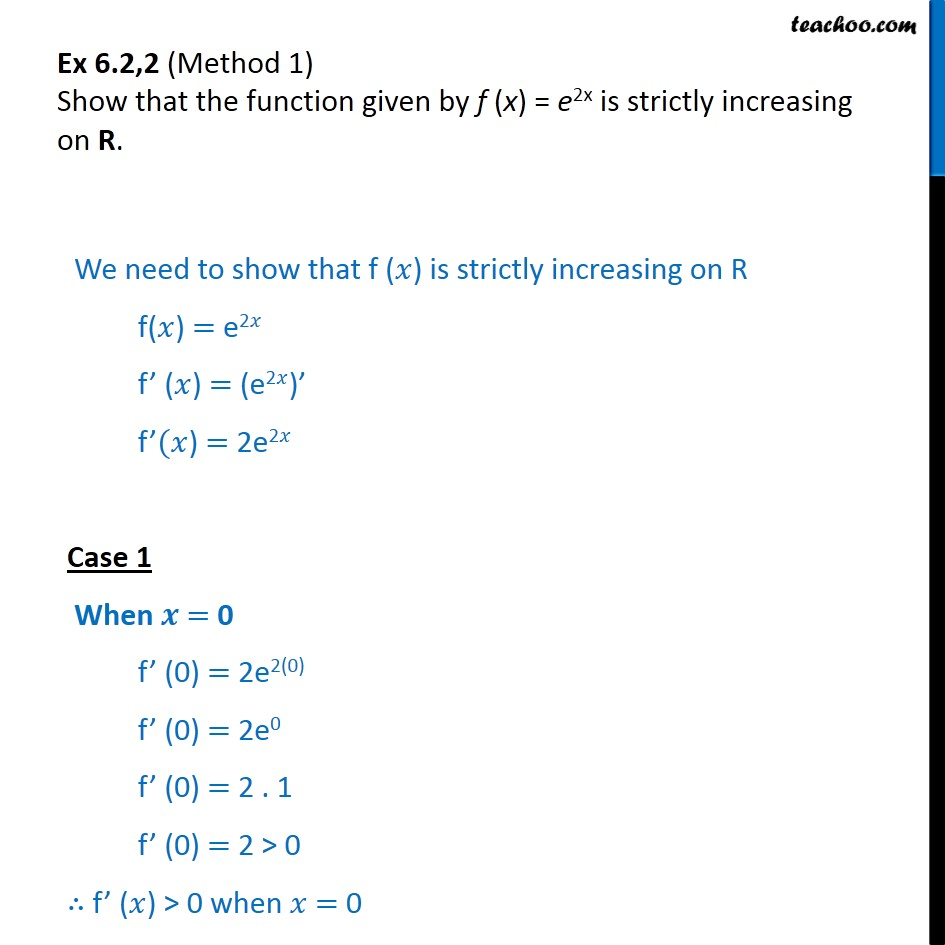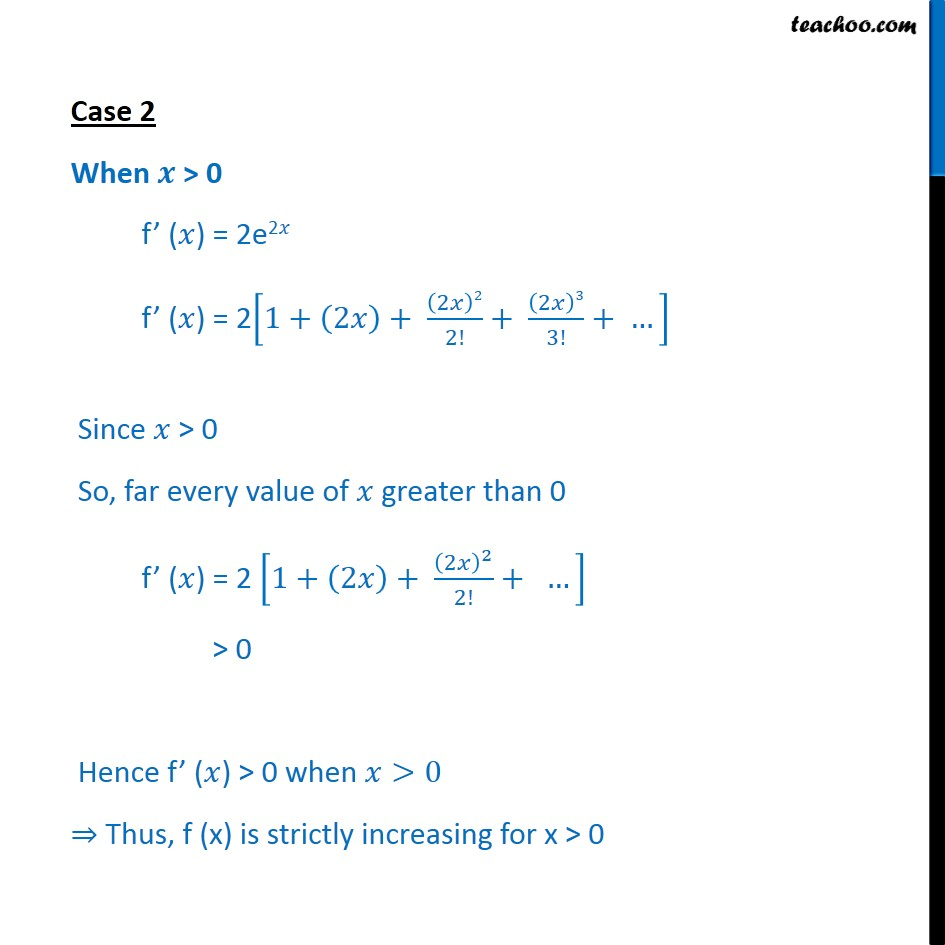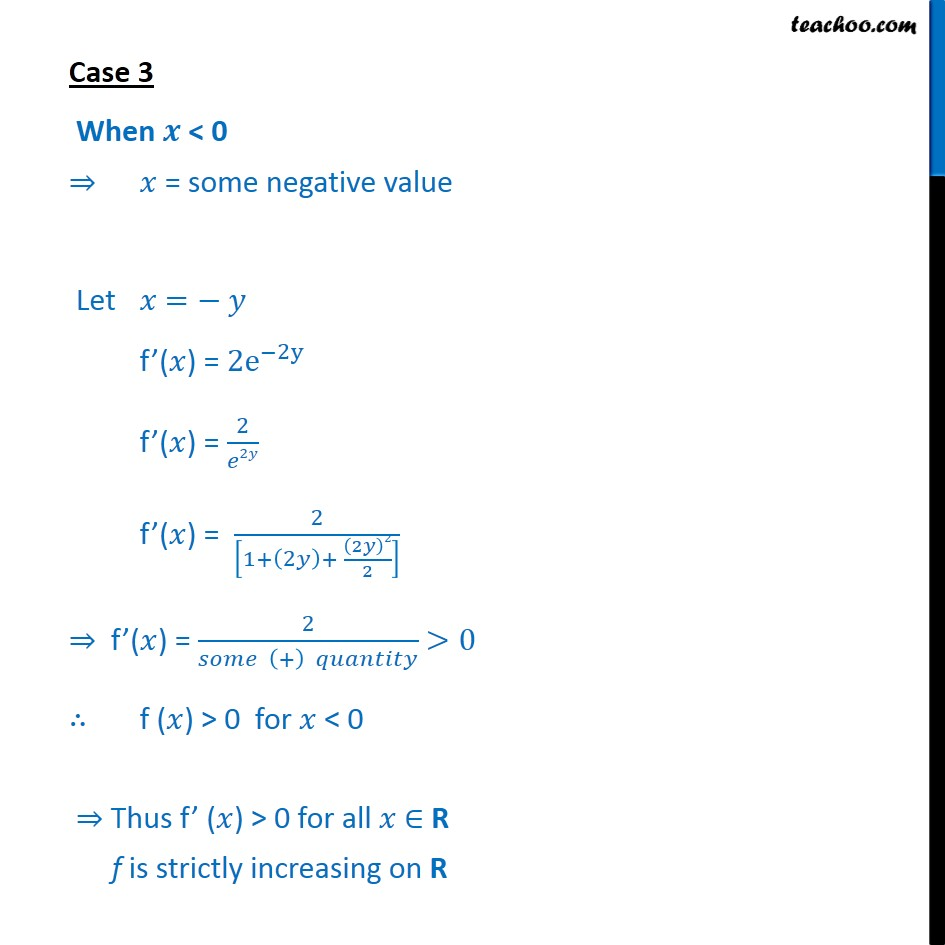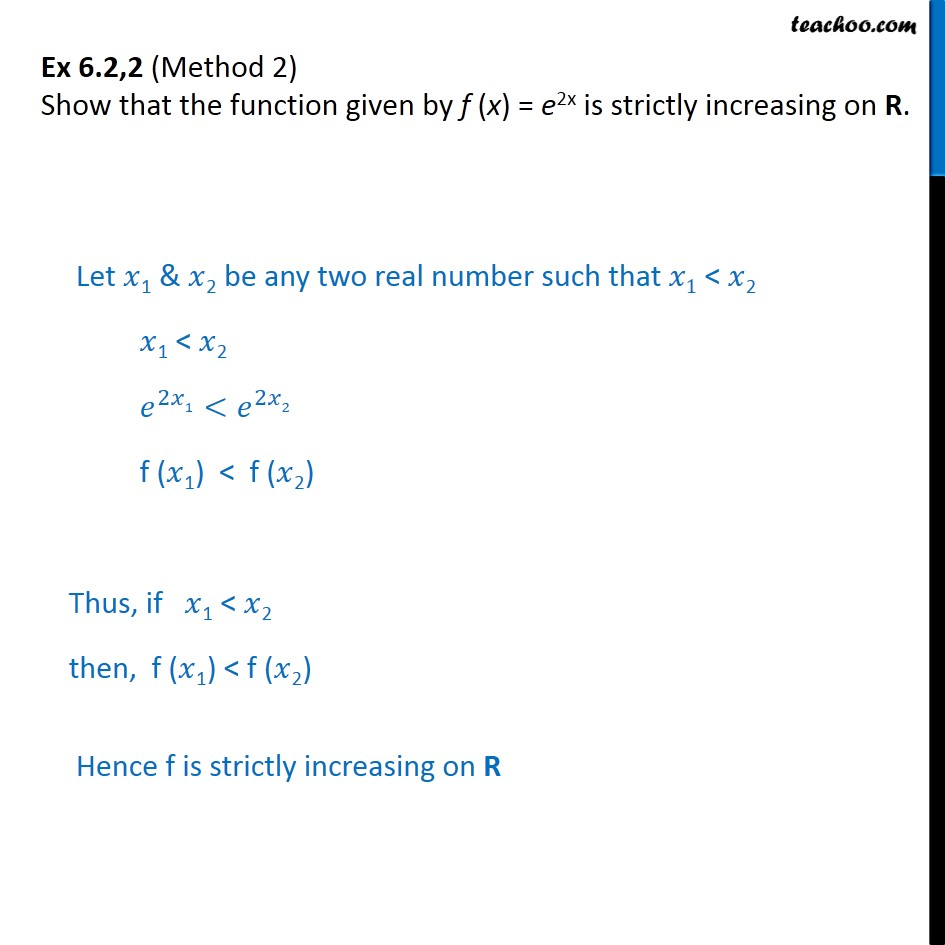Subscribe to our Youtube Channel - https://you.tube/teachoo

1. Chapter 6 Class 12 Application of Derivatives
2. Serial order wise
3. Ex 6.2

Transcript

Ex 6.2,2 (Method 1) Show that the function given by f (x) = e2x is strictly increasing on R. We need to show that f (𝑥) is strictly increasing on R f(𝑥) = e2𝑥 f’ (𝑥) = (e2𝑥)’ f’(𝑥) = 2e2𝑥 Case 1 When 𝒙 = 0 f’ (0) = 2e2(0) f’ (0) = 2e0 f’ (0) = 2 . 1 f’ (0) = 2 > 0 ∴ f’ (𝑥) > 0 when 𝑥 = 0 Case 2 When 𝒙 > 0 f’ (𝑥) = 2e2𝑥 f’ (𝑥) = 2﷐1+﷐2𝑥﷯+ ﷐﷐2𝑥﷯2﷮2!﷯+ ﷐﷐2𝑥﷯3﷮3!﷯+ …﷯ Since 𝑥 > 0 So, far every value of 𝑥 greater than 0 f’ (𝑥) = 2 ﷐1+﷐2𝑥﷯+ ﷐﷐﷐2𝑥﷯﷮2﷯﷮2!﷯+ …﷯ > 0 Hence f’ (𝑥) > 0 when 𝑥>0 ⇒ Thus, f (x) is strictly increasing for x > 0 Case 3 When 𝒙 < 0 ⇒ 𝑥 = some negative value Let 𝑥 =− 𝑦 f’(𝑥) = 2﷐e﷮−2y﷯ f’(𝑥) = ﷐2﷮𝑒2𝑦﷯ f’(𝑥) = ﷐2﷮﷐1+﷐2𝑦﷯+ ﷐﷐2𝑦﷯2﷮2﷯﷯﷯ ⇒ f’(𝑥) = ﷐2﷮𝑠𝑜𝑚𝑒 ﷐+﷯ 𝑞𝑢𝑎𝑛𝑡𝑖𝑡𝑦﷯ >0 ∴ f (𝑥) > 0 for 𝑥 < 0 ⇒ Thus f’ (𝑥) > 0 for all 𝑥 ∈ R f is strictly increasing on R Ex 6.2,2 (Method 2) Show that the function given by f (x) = e2x is strictly increasing on R. Let 𝑥1 & 𝑥2 be any two real number such that 𝑥1 < 𝑥2 𝑥1 < 𝑥2 ﷐𝑒﷮2𝑥1﷯<﷐𝑒﷮2𝑥2﷯ f (𝑥1) < f (𝑥2) Thus, if 𝑥1 < 𝑥2 then, f (𝑥1) < f (𝑥2) Hence f is strictly increasing on R

Ex 6.2